Reach Us+44-1522-440391
A Simple Method for Estimating Confidence Intervals for Exposur e Adjusted Incidence Rate and Its Applications to Clinical Trials | OMICS International
Journal of Biometrics & Biostatistics

Like us on:

All submissions of the EM system will be redirected to Online Manuscript Submission System. Authors are requested to submit articles directly to Online Manuscript Submission System of respective journal.

# A Simple Method for Estimating Confidence Intervals for Exposur e Adjusted Incidence Rate and Its Applications to Clinical Trials

Xin He1*, Li Chen2, Lei Lei2, H. Amy Xia2 and Mei-Ling Ting Lee1

1University of Maryland, College Park, MD, USA

2Amgen Inc, Thousand Oaks, CA, USA

*Corresponding Author:
Xin He
Department of Epidemiology and Biostatistics
2234H SPH Building, School of Public Health
University of Maryland, College Park, MD 20742, USA
Tel: 301-405-2551
Fax: 301-314-9366
E-mail: [email protected]

Received Date: June 06, 2014 Accepted Date: June 23, 2015 Published Date: June 30, 2015

Citation: He X, Chen L, Lei L, Xia HA, Lee MLT (2015) A Simple Method for Estimating Confidence Intervals for Exposur Adjusted Incidence Rate and Its Applications to Clinical Trials. J Biom Biostat 6:238. doi:10.4172/2155-6180.1000238

Copyright: © 2015 He X, et al. This is an open-access article distributed under the terms of the Creative Commons Attribution License, which permits unrestricted use, distribution, and reproduction in any medium, provided the original author and source are credited.

Visit for more related articles at Journal of Biometrics & Biostatistics

#### Abstract

Assessment of drug safety typically involves estimation of occurrence rate of adverse events. Most often, the crude percentage (subject incidence) is used to estimate adverse event rate. However, in some situations, the exposure adjusted incidence rate (EAIR) may be a more appropriate measure to account for the potential difference in the duration of drug exposure or the follow-up time among individuals. In this article, we establish the asymptotic properties of the EAIR under certain assumptions, and propose a general and simple approach for variance estimation and for calculating the confidence interval of the rate. Simulation studies are conducted to evaluate the performance of the proposed approach. The results show that the proposed procedures perform well for various scenarios of different follow-up patterns. Data from a clinical trial are used to demonstrate the application of the method. A SAS macro is provided in the appendix.

#### Introduction

In safety analyses in clinical trials, our main interest is to describe the pattern of occurrence of adverse events and to use sound statistical approaches to estimate the rate of occurrence. A desirable measurement should have good properties on the rate of occurrence and convey the risk associated with the adverse event in order to facilitate risk and benefit assessment in decision making. Several measurements have been used to estimate rates of occurrence of adverse events associated with exposure to a drug. The crude percentage is the most commonly used measurement for summarizing safety data. It is defined as the number of subjects exposed to the drug and experiencing a certain event divided by the total number of subjects exposed to the drug, regardless of duration of follow-up. The crude percentage is most appropriate where all subjects are treated and followed for the same period of time, for very short-term drug exposure, or for acute events following close in time after exposure. In situations where subjects have different durations of drug exposure, or a long-term follow-up, the crude percentage is not appropriate because it does not take into consideration of the duration of drug use . To adjust for potential differences on duration of drug exposure, the exposure adjusted incidence rate (EAIR), which is also referred to as incidence density, may be used. It is defined as the number of subjects exposed to the drug and experiencing a certain event divided by the total exposure time of all subjects who are at risk for the event. Specifically, for subjects with no event, the exposure time is the time from the first drug intake to the last follow-up assessment; for subjects with at least one event, the exposure time is the time from the first drug exposure to first event. The EAIR is a measure of average events per unit time of exposure or follow-up. The underlying assumption with this measure is that the risk of an event occurring is constant over time. In other words, assuming that the occurrences of a specific event are independent and have a constant hazard rate over the duration of the study, the EAIR is most appropriate to estimate the occurrence rate. In the situations where the occurrences of adverse events are delayed, or the risk associated with an event varies over time, the EAIR would not be an appropriate measure . In these situations, the cumulative rate based on timeto- event analysis is most appropriate because it makes no assumption about the underlying risk per unit of time and takes into consideration of different durations of exposure and when the events occur relative to the number of subjects at risk.

The development and application of statistical methods on assessing safety rates in clinical trials has been relatively limited because historically the assessment of safety data has not received the same level of attention as that for efficacy. While the EAIR is advantageous over the crude percentage by accounting for various exposure or follow-up times among subjects in clinical trials, more precise estimates are important to characterize the safety profile of a product and to afford more precise confidence intervals for comparative purposes. Among others, Koch et al.  discussed the application of incidence density, or the EAIR, to drug safety data, and developed a Mantel-Haenszel procedure to test the association between treatment and event occurrence based on a Poisson distribution assumption. LaVange et al.  investigated the ratio estimation method for incidence density via a first-order Taylor series approximation and estmiated the adjusted incidence density ratios using model-based approaches. Tangen and Koch  proposed nonparametric covariance methods for comparing two treatments for incidence densities across multiple intervals, while Saville et al.  further extended such nonparametric methods in settings where subjects may experience multiple events. Besides using the incidence density ratio (EAIR ratio) to measure the relative risk between two groups, another commonly used measure is the risk difference (EAIR difference). As noted in Liu et al. , the choice of relative risk or risk difference is somewhat arbitrary, but the EAIR difference is more appropriate for rare adverse events where the ratio may not be defined if there are no events in the reference group. They  investigated the appropriateness of the crude percentage versus the EAIR under various scenarios and reviewed four approaches of constructing confidence intervals for the difference of two EAIRs under the Poisson distribution assumption, such as Wald’s method, the two-by-two table method, the Miettinen and Nurminen (MN) method , and the conditional MN method. They performed extensive simulation studies and concluded that 1) in general the crude percentage may be biased especially when the time to event may be censored prior to the maximum follow-up time; 2) the EAIR performs well when the hazard is constant regardless of censoring; 3) the MN method outperforms the other three methods in terms of the coverage probability. However, the MN method does not directly estimate the variance of the EAIR for individual groups and it is computationally intensive. In this article, we propose a general and simple approach for variance estimation and for calculating the confidence interval of the rate. The main advantages of our approach include the closed form for the estimation of the variance of the EAIR in lieu of the MN method involving numerical iterative procedures.

The remainder of this article is organized as follows. In Section 2, the asymptotic properties of the EAIR in one group are established and evaluated by simulation studies. In Section 3, the variance estimate for the rate and the confidence interval of the rate difference between two groups are calculated and simulation studies are conducted to compare our method with the MN method. Section 4 applies the proposed method of the EAIR to data from an integrated clinical evaluation of safety and Section 5 concludes with discussions and closing remarks.

#### 2. Exposure Adjusted Incidence Rate (EAIR) in One Group

2.1 Definition of EAIR

Let ti denote time to first event for subject i, and li denote the follow-up time for subject i, then the exposure adjusted incidence rate (EAIR) can be defined as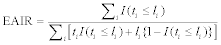or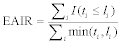2.2 General Method

Asymptotic Properties: Suppose that ti follows an exponential distribution with parameter λ, where λ is the constant hazard for the time to first event. Define ai = I (ti ≤ li) and a = E(ai) = P (ti ≤ li), and denote bi = min(ti, li) and b = E(bi). Let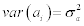,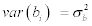and and cov(ai, bi) = ρσaσb. Assuming that both ai’s and bi’s are independent and identically distributed, respectively, by the Weak Law of Large Numbers and Central Limit Theorem, we show in Appendix A that the asymptotic distribution of the EAIR is given by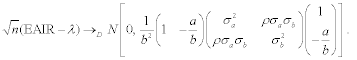(1)

Since there is no distribution assumption on li, the variance of the above normal distribution in (1) can be consistently estimated by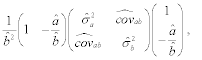(2)

where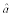and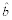ai = I (ti ≤ li) and bi = min(ti,li),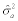and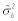are the corresponding sample variances, and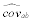is the sample covariance of ai and bi. In addition, a SAS macro is provided in Appendix B to calculate the EAIR as well as the proposed standard error and 95% confidence interval for λ.

Simulation Studies: This section reports results obtained from simulation studies conducted to assess the performance of the proposed method. The time to first event ti was assumed to follow an exponential distribution with parameter λ, which indicates the constant hazard for the time to event. Thus a small value of λ corresponds to a relatively rare event and a large value of λ corresponds to a frequent event. It was assumed that the time to early termination si is independent of ti and follows a Weibull distribution, then the density function can be expressed as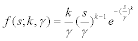for s ≥ 0, with shape parameter k > 0 and scale parameter γ > 0. The value of k determines the shape of the Weibull density and the parameter γ scales the s variable. The Weibull distribution was used in the simulation studies due to its flexibility, and it can mimic the behavior of many other statistical distributions. When k < 1, the resulting hazard (i.e., early termination rate) decreases over time; when k = 1, it results in a constant hazard; when k > 1, the resulting hazard increases over time. Let m be the maximum follow-up time, then the exposure time li = m if si ≥ m, and li =si (early termination) if si < m. Without loss of generality, we assumed that m = 1. The results given below are based on n = 200 and 400, with 10,000 replications.

Table 1 presents the simulation results obtained with λ = 0.05, 0.2, or 5; k = 0.5, 1, or 2; and γ = 0.5, or 5. The table includes the estimated relative bias in percentage (Relative Bias) given by (Average EAIR − λ)/λ × 100%, the sample standard error (SSE), the average of the standard error estimates (SE), and the empirical 95% coverage probability (CP) for λ. It can be seen that the EAIR appears to be an unbiased estimate of λ and the SSE and SE for the proposed general method are comparable, suggesting that the proposed variance estimate is reasonable. For a rare event with small λ’s (i.e., λ = 0.05), when the sample size is not large enough (i.e., n = 200), there are many replications with no information observed for the event, thus the corresponding coverage probabilities of both methods are below 95%. However, when the sample size increases to 400, the coverage probability improves to be closer to 95%. Under the Weibull distribution, when γ increases, the mean value of the generated time to early termination si increases, resulting in a more precise estimate of λ. This is evidenced in Table 1. In addition, further simulation studies were conducted to compare our proposed approach with Wald’s method which is another normal approximation approach based on the Poisson distribution assumption with the estimated variance of EAIR given by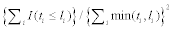.Our proposed method provided a slightly more accurate coverage probability compared to Wald’s method in most scenarios (data not shown).

General Method
Sample Size λ k γ Relative Bias SSE SE CP
200 0.05 0.5 0.5 0.60% 0.0255 0.0251 0.9041
5 -0.13% 0.0186 0.0186 0.9231
1 0.5 -0.33% 0.0243 0.0244 0.9174
5 0.36% 0.0169 0.0169 0.9329
2 0.5 0.64% 0.0239 0.0242 0.93
5 -0.30% 0.0162 0.0162 0.9115
0.2 0.5 0.5 -0.24% 0.0516 0.0515 0.9336
5 0.54% 0.039 0.0386 0.9429
1 0.5 0.72% 0.0505 0.0502 0.9375
5 0.27% 0.0349 0.035 0.9425
2 0.5 -0.07% 0.049 0.0491 0.9373
5 0.28% 0.0336 0.0336 0.9442
5 0.5 0.5 0.72% 0.4661 0.4625 0.9488
5 0.63% 0.3909 0.3892 0.9486
1 0.5 0.51% 0.422 0.4213 0.9501
5 0.57% 0.3658 0.364 0.9487
2 0.5 0.41% 0.3936 0.3941 0.9481
5 0.53% 0.3596 0.3578 0.9486
400 0.05 0.5 0.5 -0.28% 0.0176 0.0176 0.9187
5 0.12% 0.013 0.0131 0.939
1 0.5 0.35% 0.0172 0.0172 0.9234
5 0.03% 0.0119 0.0119 0.935
2 0.5 0.31% 0.0169 0.017 0.9306
5 0.16% 0.0113 0.0114 0.9415
0.2 0.5 0.5 0.30% 0.0362 0.0364 0.9433
5 -0.05% 0.0269 0.0272 0.9456
1 0.5 0.05% 0.0354 0.0353 0.9417
5 0.06% 0.0247 0.0247 0.946
2 0.5 0.18% 0.0349 0.0347 0.9426
5 0.22% 0.0235 0.0237 0.9483
5 0.5 0.5 0.23% 0.3256 0.3254 0.95
5 0.16% 0.2728 0.274 0.9509
1 0.5 0.13% 0.2952 0.2968 0.9498
5 0.16% 0.2572 0.2563 0.9496
2 0.5 0.24% 0.2778 0.2781 0.9491
5 0.22% 0.2507 0.2518 0.9496

Table 1: Simulation results of EAIR for the proposed general method in Subsection 2.2.

In these following two subsections, we consider special cases where assumptions on the distribution of the follow-up time can be made.

2.3 Distribution-Specific Case: Scenario 1

Assumption: Consider the following distribution of follow-up time li: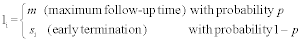where the maximum follow-up time m is fixed and si follows a uniform distribution between 0 and m, representing the situation that early termination times are evenly distributed.

Asymptotic Properties: Analogous to the general method in the previous subsection, we have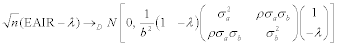(3)

where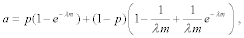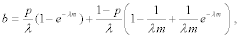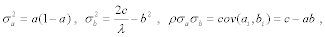and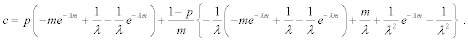Besides the general method in the previous subsection, another way to estimate the variance is based on consistent estimates of λ and p, which are given by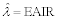and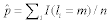Although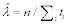is a more consistent estimate of λ, it cannot be applied since not all ti’s would be observed in reality.

Simulation Studies: Simulation studies were conducted to compare the performances between the general method and the distributionspecific method in this scenario. The time to first event ti was assumed to follow an exponential distribution with parameter λ. The follow-up time li = m (maximum follow-up time) with probability p and li = si (early termination) with probability 1 − p. Here si was assumed to be independent of ti and follow a uniform distribution between 0 and m.Without loss of generality, we assumed that m = 1. The results given below are based on n = 200 and 400, with 10, 000 replications.

Table 2 presents the simulation results obtained with λ = 0.05, 0.2, or 5 and p = 0.2, 0.8, or 1. When p = 1, there is no early termination and all subjects have the same follow-up time li = m. SE and CP are corresponding to the average of the standard error estimates and the empirical 95% coverage probability for λ for each method in this scenario. It can be seen that EAIR seems to be an unbiased estimate of λ and both standard error estimates seem quite close to the sample standard error, suggesting that both proposed variance estimates are reasonable. The table also shows that the results become better when the sample size increases.

Specific Method Distribution Specific Method
Sample Size λ p Relative Bias SSE SE CP SE CP
200 0.05 0.2 0.47% 0.0207 0.0208 0.9274 0.0207 0.9286
0.8 -0.28% 0.0169 0.0169 0.9321 0.0169 0.9319
1 -0.35% 0.016 0.016 0.9186 0.016 0.9188
0.2 0.2 0.12% 0.0421 0.0426 0.9436 0.0425 0.9431
0.8 0.49% 0.0351 0.0352 0.946 0.0351 0.9463
1 0.40% 0.0336 0.0334 0.9443 0.0333 0.9429
5 0.2 0.55% 0.3867 0.3881 0.9481 0.3887 0.9514
0.8 0.52% 0.364 0.3641 0.9473 0.3646 0.952
1 0.47% 0.3584 0.3569 0.9459 0.3573 0.948
400 0.05 0.2 0.10% 0.0144 0.0146 0.9408 0.0146 0.9421
0.8 -0.09% 0.0118 0.0119 0.9356 0.0119 0.9351
1 -0.32% 0.0113 0.0113 0.9417 0.0113 0.9419
0.2 0.2 -0.19% 0.0298 0.03 0.9463 0.03 0.9463
0.8 0.31% 0.0246 0.0248 0.9497 0.0248 0.9498
1 0.17% 0.0235 0.0236 0.95 0.0235 0.95
5 0.2 0.29% 0.2779 0.2737 0.9467 0.2739 0.9476
0.8 0.27% 0.2584 0.2567 0.9484 0.2568 0.9516
1 0.32% 0.2508 0.2516 0.9498 0.252 0.951

Table 2: Simulation results of EAIR for distribution-specific case in Subsection 2.3.

2.4 Distribution-Specific Case: Scenario 2

Assumption: Consider the following distribution of follow-up time li: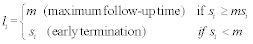where si, early termination time, follows an exponential distribution with parameter β.

Asymptotic Properties: Analogous to Scenario 1, it can be shown that in the asymptotic expression (3),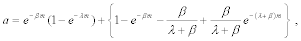where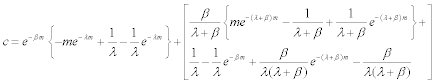Besides the general method, another way to estimate the variance is based on consistent estimates of λ and β , which are given byand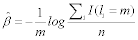Simulation Studies: Simulation studies were also conducted to compare the performances between the general method and the distribution-specific method in this scenario. It was assumed that si follows an exponential distribution with parameter β, then the follow-up time li = m (maximum follow-up time) if si ≥ m, and li = si (early termination) if si < m. Without loss of generality, we assumed that m = 1. The results given below are based on n = 200 and 400, with 10,000 replications.

Table 3 presents the simulation results obtained with λ = 0.05, 0.2, or 5 and β = 0.05, 0.5, or 1. SE and CP are corresponding to the average of the standard error estimates and the empirical 95% coverage probability for λ for each method in this scenario. As shown in Table 3, the EAIR seems to be an unbiased estimate of λ. The general method and the distribution-specific method give very similar results in terms of standard error estimate and coverage probability. The table also indicates that the results tend to be better with a greater sample size.

Specific Method Distribution Specific Method
Sample Size λ p Relative Bias SSE SE CP SE CP
200 0.05 0.05 0.26% 0.0162 0.0163 0.9081 0.0163 0.9076
0.5 0.18% 0.0182 0.0181 0.926 0.0181 0.9268
1 0.20% 0.0202 0.0202 0.9228 0.0202 0.9212
0.2 0.05 0.25% 0.0334 0.0338 0.9455 0.0337 0.9446
0.5 0.44% 0.0375 0.0376 0.9444 0.0375 0.9439
1 0.31% 0.0414 0.0417 0.9398 0.0416 0.9382
5 0.05 0.60% 0.359 0.3589 0.9465 0.3595 0.9495
0.5 0.59% 0.3774 0.3745 0.948 0.3747 0.9493
1 0.32% 0.3909 0.3896 0.9464 0.39 0.9502
400 0.05 0.05 0.09% 0.0114 0.0115 0.935 0.0115 0.935
0.5 -0.06% 0.0128 0.0128 0.9331 0.0128 0.9327
1 0.26% 0.0142 0.0143 0.9301 0.0142 0.9285
0.2 0.05 0.08% 0.0238 0.0238 0.9523 0.0238 0.952
0.5 -0.02% 0.0266 0.0264 0.943 0.0264 0.9434
1 0.29% 0.0291 0.0294 0.9488 0.0294 0.9483
5 0.05 0.19% 0.2583 0.2527 0.9446 0.2529 0.947
0.5 0.20% 0.2624 0.2633 0.9503 0.2636 0.9518
1 0.18% 0.2755 0.2744 0.9491 0.2751 0.9493

Table 3: Simulation results of EAIR for distribution-specific case in Subsection 2.4.

#### 3. Confidence Interval for the EAIR Difference: A Comparison with the MN Method

Without loss of generality, we consider a study of two independent treatment groups. Let λ1 and λ2 be the constant hazard rate in each of the two groups. A 100% × (1 − α) confidence interval for λ1 − λ2 is given by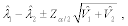where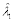and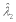are the estimated EAIRs,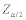is the 100(1 − α/2) th percentile of a standard normal random variable, and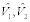represent the corresponding estimated variances as defined in (2).

Liu et al.  investigated four approaches to construct confidence intervals for the EAIR difference between two treatment groups. In their simulation studies, the time to first event of interest was assumed to follow an exponential distribution with parameter λ, which is a special case of Subsection 2.3 in this article when p = 1 (no early termination). Two hundred subjects were randomized evenly to one of the two treatment groups, and the simulations were performed for λ1 = 0.02, 0.2, 1, and 5 (for group 1) and λ2 = 0.002 − 0.1 by 0.002, 0.1 − 1 by 0.02, and 1 − 5 by 0.2 (for group 2). Based on their results, the MN method was the most accurate one in terms of coverage probability among the four approaches, especially when λ1 and λ2 were small (λ1 = 0.02 and λ2 = 0.002 − 0.2).

At this particular setup of λ1 and λ2 with p = 1 and m = 1, simulation studies were conducted to compare the performances between the MN method and our proposed general method described in Subsection 2.2 based on 10,000 replications. There are two reasons that we used the general method instead of the distribution-specific method to do the comparison. The first one is that the MN method and the general method are more comparable, since neither of them requires any distributional assumption of the follow-up time li. The other one is that as shown in Table 2, the general method works similarly as the distribution-specific method. Based on Figure 1 (n = 200 per group) and Figure 2 (n = 400 per group), it is clear that both methods have similar performance in terms of coverage probability. We also did simulation studies for boarder values of λ1 and λ2, and obtained similar results. However, the MN method involves lots of equation solving and iterations and thus is much more computationally intensive (i.e., around 50 times slower in terms of computational speed when n = 200 per group). When the sample size is large, our proposed method is more desirable due to its simplicity and computational efficiency.

Figure 1: Empirical probability of coverage for 95% confidence intervals (based on 10,000 simulations, n=200 per group).

Figure 2: Empirical probability of coverage for 95% confidence intervals (based on 10,000 simulations, n=400 per group).

#### 4. An Illustrative Example

To illustrate the methods given in the previous sections, we applied them to the analysis of adverse events in an integrated clinical evaluation of safety. Subject level adverse event records collected in five clinical trials were included in the analysis. Three of them, dated 2003 and 2004, were designed as half-year long efficacy studies with safety evaluation being the secondary objective. The remaining two, dated 1997 and 2004, were designed as one-year long safety studies with the incidence rate of adverse events being the primary endpoint. We treated adverse event data from the first three studies as from a “short study”, and those from the other two as from a “long” one. A total of 332 patients were in the short study, where the planned study length was 32 weeks. For those 483 patients in the long study, the planned study length was 52 weeks. The primary objective was to compare the rates of adverse events between the two studies.

Table 4 presents the crude percentages and the EAIRs for three selected adverse events. In the table, N is the total number of patients and n* represents the number of patients who have experienced the event. The 95% confidence intervals for the crude percentage differences were computed based on binomial assumption  and the 95% confidence intervals for the EAIR differences were calculated according to our proposed method (95% CIP) in Subsection 2.2 and the MN method (95% CIMN). The results for the event “cough” suggest that the differences of both crude percentages and EAIRs were not significant between the short and long study groups. However, the results for “diarrhea” indicate that the long study group had a significantly greater crude percentage than the short study group, but the EAIR difference was not significant. The conclusions regarding “arthralgia” are consistent with those of “diarrhea”. Furthermore, our proposed method and the MN method produce fairly similar confidence intervals for all three EAIR differences.

Crude Percentage EAIR
Short N=322 n* LongN=483n* Short (SE) Long (SE) Difference (95% CI) Short(SE) Long(SE) Difference
Adverse Event (95% CIP) (95% CIMN)
Diarrhea 34 102 0.1056 0.2112 0.1056 0.2501 0.3105 0.0604
-0.0171 -0.0186 (0.0561,0.1551) (-0.0432) (-0.0302) (-0.0428, 0.1636) (-0.0524, 0.1731)
Cough 26 50 0.0807 0.1035 0.0228 0.1898 0.1431 -0.0467
-0.0152 -0.0139 (-0.0176,0.0632) (-0.037) (-0.0201) (-0.1293, 0.0360) (-0.1223, 0.0290)
Arthralgia     0.059 0.1284 0.0694 0.1364 0.1808 0.0444
Arthralgia 19 62 -0.0131 -0.0152 (0.0301, 0.1087) (-0.0313) (-0.0228) (-0.0316, 0.1204) (-0.0413, 0.1301)

Table 4: Comparisons of crude percentages and EAIRs for the clinical trial example.

To visually check the required assumptions of the EAIR and the conclusions above, survival analysis was applied to those three adverse events. Figure 3 displays the Nelson-Aalen cumulative hazard estimate for each adverse event. Since the main parts of those cumulative hazard estimates are approximately straight lines, the constant hazard assumption seems reasonable. Moreover, the graphic results from survival analysis are consistent with the estimates from the EAIR.

#### 5. Discussion and Concluding Remarks

In this article, asymptotic properties of exposure adjusted incidence rate are established under certain assumptions, and a general and simple approach is proposed for variance estimation and for calculating the confidence interval of the rate. A key feature of the approach is that it is easy to implement and interpret. As shown in the simulation studies for the EAIR in one group and the EAIR difference between two groups, the proposed method is unbiased with good coverage probabilities and more efficient in computation time in contrast to other existing methods as it does not involve intensive numerical iterations. Moreover, it is also robust in various scenarios with different follow-up time distributions.

Note that the proposed method may provide less than 95% coverage for rare events when the sample size is not large enough. As a direction for future research, it would be useful to investigate the use of continuity correction [9,10] or log-transformed EAIR to improve the normal approximation for small samples.

When summarizing adverse events in safety analyses using the EAIR, it is important to check the constant hazard (exponential distribution) assumption. If this assumption is violated, the EAIR may not be an appropriate statistical measure to evaluate adverse events. Alternative survival analysis may be considered. The life table method or Kaplan-Meier estimator could be more suitable for noncontact hazard situations . When the study interest is generalized into the recurrent event process, several approaches have been proposed using the intensity function with Poisson assumption in the framework of counting processes , the mean function without Poisson assumption [12,13], or the mean cumulative function .

#### Acknowledgements

We wish to thank the editor and two reviewers for their thoughtful and valuable comments and suggestions.

#### Appendix A: Proof of the Asymptotic Properties of the EAIR

By Weak Law of Large Numbers,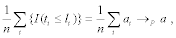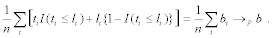Moreover,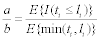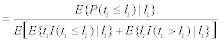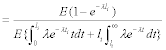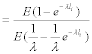= λ .

Let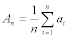and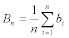where (ai, bi) are i.i.d,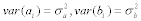and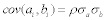.Now consider the asymptotic distribution of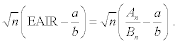By Central Limit Theorem, we have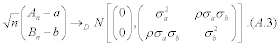Since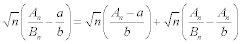and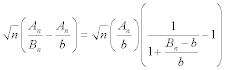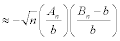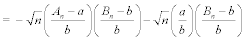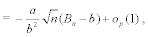we have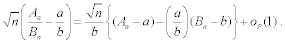Therefore,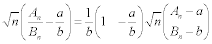yielding that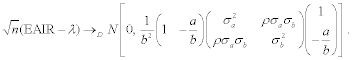Appendix B: SAS Macro to Calculate the EAIR and Its 95% Confidence Interval

%MACRO eair(data,time,event);

/* data: dataset name (contains time, event)

time: exposure time

event: event indicator (1-yes, 0-no)*/

DATA one;

SET &data;

t=&time;

indicator=&event;

RUN;

PROC IML;

USE one;

n=nrow(x);

EAIR=sum(x[,2])/sum(x[,1]);

a=mean(x[,2]);

b=mean(x[,1]);

c=cov(x);

d=-a/b;

SE=SQRT((c[2,2]+2*d*c[1,2]+d*d*c[1,1])/b/b/n);

EAIR_LL=EAIR-1.96*SE;

EAIR_UL=EAIR+1.96*SE;

CREATE RESULT VAR {EAIR SE EAIR_LL EAIR_UL};

APPEND;

CLOSE RESULT;

QUIT;

DATA RESULT;

SET RESULT;

LABEL EAIR="Estimated EAIR"

SE="Standard Error"

EAIR_LL="95% CI Lower Limit"

EAIR_UL="95% CI Upper Limit";

RUN;

PROC PRINT DATA=RESULT LABEL NOOBS;

VAR EAIR SE EAIR_LL EAIR_UL;

RUN;

%MEND eair;

#### References

Select your language of interest to view the total content in your interested language

### Article Usage

• Total views: 13697
• [From(publication date):
August-2015 - Jul 19, 2019]
• Breakdown by view type
• HTML page views : 9791Can't read the image? click here to refresh